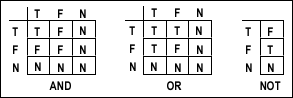Theseus Research : Technical Papers : NULL Convention Logic™ Page 3 of 26
2. Making Boolean Logic Symbolically Complete

The invocation model teaches that to achieve symbolic completeness of expression, both data transformation and control must be expressed in the most primitive inherently enforced mutually exclusive value assertion domain of the expression. In a mutually exclusive value assertion domain, only one of a set of two or more values can be asserted at a time. Every expression environment has a primitive mutually exclusive value assertion domain. For Boolean logic the primitive mutually exclusive value assertion domain consists of True and False, only one of which can be asserted at a time. For the decimal number system the values 0 through 9 are mutually exclusive. In both of these cases the values comprising the mutually exclusive assertion domain express only data meanings. There is no value in either domain that expresses a control or non-data meaning. The Invocation Model teaches that to make Boolean logic symbolically complete, we must add a value to the primitive mutually exclusive assertion domain.

Making Boolean logic symbolically complete will be presented in two steps. The first step is to assign a value in the most primitive mutually exclusive assertion domain to express the validity/invalidity of data. To retain the sense of Boolean logic, we will retain the two values expressing data meanings in the primitive domain, so the first step is to add a third value to the mutually exclusive value assertion domain which we will call NULL (not a valid data value). We then define the truth tables to assert a data value (True, False) only when both input values are data values and to assert a NULL value otherwise. Figure 1 shows the NULL value added to the traditional Boolean truth tables and specifies the behavior of these preliminary NULL Convention Logic gates.Figure 1. Boolean truth tables with NULL value added.

We now have a three value logic. Each wire can assert one of three values (T, F or N) and each gate resolves three input values. The values T and F will be collectively referred to as data values and NULL will be referred to as a non-data value. If a data value is asserted on a wire, the data value is valid. A transition from NULL to a data value marks the beginning of validity. A transition from a data value to NULL marks the end of validity, or the beginning of invalidity.

These truth tables enforce the completeness of input criteria for data. This means that a gate will assert a data result value only when a complete set of data values is present at its input. In this case, a complete set of input data values is for both inputs to be data (either TRUE or FALSE, but not NULL). Furthermore, the asserted result data value is the correct resolution of the presented input data values if either or both input values are NULL, a NULL result value is asserted.